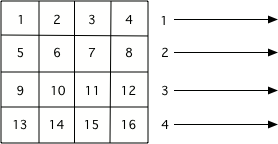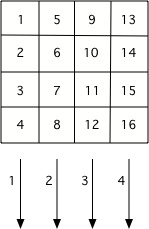# 18.5. Changing Step 6: Changing where we put the colors¶

We have been getting a list of all of the pixels from an image using img.getPixels() and then looping through all the pixels using for p in pixels:. This processed all the pixels in the first row, then all the pixels in the second row, and so on until it processed all the pixels in the image.Figure 1: The order that pixels are processed if you use img.getPixels()¶

We can also loop through all the x and y values in the image and then use the current (x,y) location to get the pixel from the image. This approach uses two for loops with one inside of the other. This is called a nested loop. See step 3 below for the nested loop. Also, notice that we now need to get the pixel at the (x,y) location in step 4 as well.

Since the outer loop changes the x (the column) and the inner loop changes the y (the row), we will be processing all the pixels in the first column, then all the pixels in the second column, and so on until we process all the pixels in the image. The order doesn’t matter if we are just setting the red to zero at every pixel as shown below.Figure 2: The order that pixels are processed if you use a nested loop with x changing in the outer loop and y changing in the inner loop.¶

We don’t always have to modify the pixel color. Sometimes we can just change the color at a different (x,y) location than the current pixel. This time we will skip step 5 (changing the color) and change step 6 to get the pixel at (x,y) and copy the color from that pixel to the pixel at location (y,x). So this will copy the color from (0,2) to (2,0). Later it will also copy the color from (2,0) to (0,2), since this loops through all the pixels in the image, but that will just copy back the original color.

Try the program above on some of the other images as well by changing the file name on line 5. Does it always have the same effect?

This one does a little math with the x and y.

Try the program above on some of the other images as well by changing the file name on line 5. Which picture looks the best after you run the program?